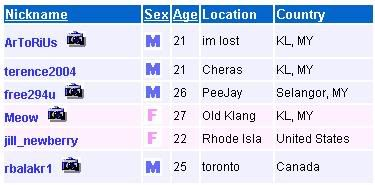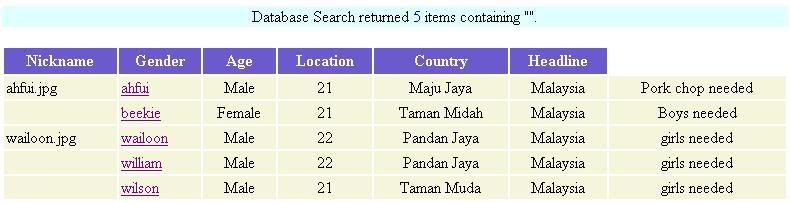# statement inside array

A

#### Anonymous

##### Guest
Code:
``````while (\$number_of_array = mysql_fetch_array(\$query)) {

echo "<TD><a href=viewuser.php?name=\$number_of_array[nickname] target=_blank>\$number_of_array[nickname]</a></TD>\n";
echo "<TD>\$number_of_array[gender]</TD>\n";
echo "<TD>\$age</TD>\n";
echo "<TD>\$number_of_array[location]</TD>\n";
echo "<TD>\$number_of_array[country]</TD>\n";
}``````

can i put the if else statement or while loop behind:
<a href=viewuser.php?name=\$number_of_array[nickname] target=_blank>\$number_of_array[nickname]</a>

if the value is equal to 1, then echo a picture

something like the picture attached...i've tried, but got error...

thanks.

Could you rephrase your question? If you just want to show an image if a particular condition is true, then test for that condition (with if) and if it's true, output the code for the image, and if not, don't.

in my table has 1 column named "image", default is 0.
when i upload the picture, the 0 will set to 1.
then check the value, if the value is equal to 1, then show the camera.gif
this is what i meaned...

Okay, so have an if statement that checks whether it's set to 1. If it is, display the image. If it isn't, don't.

Code:
``````while (\$number_of_array = mysql_fetch_array(\$query)) {
echo "<td>";
if(\$number_of_array['image']) echo \$number_of_array['image_name'];
echo '</td>';
echo "<TD><a href=viewuser.php?name=\$number_of_array[nickname] target=_blank>\$number_of_array[nickname]</a></TD>\n";
echo "<TD>\$number_of_array[gender]</TD>\n";
echo "<TD>\$age</TD>\n";
echo "<TD>\$number_of_array[location]</TD>\n";
echo "<TD>\$number_of_array[country]</TD>\n";
}``````

That what you mean?

this is the result:Do you want me to hold your hand while doing this?

I gave you an idea on how to do it.. you will have to tweak it to your liking